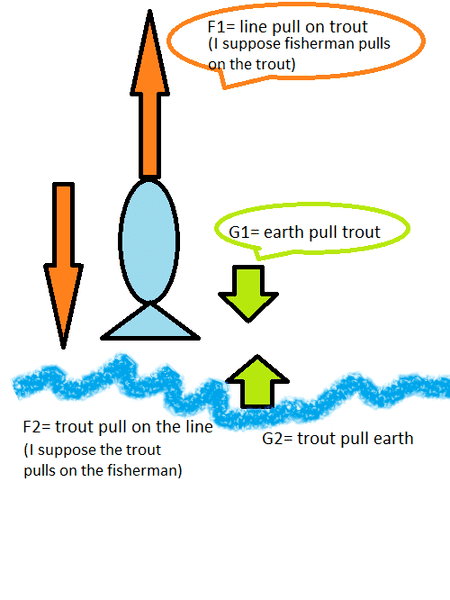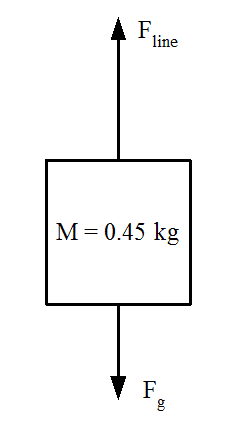# Newtonian mechanics; word problem; calculate forces

## Homework Statement

Word problem.

Fisherman lifts a trout with his fishing rod directly upwards. The fish is a trout. The trout's mass is 0,45Kg. The trout's acceleration is 2,5m/s^2.

a) draw free body diagram for the trout
b) with how great a force, does the fishing line affect the trout?

Newton's laws

F=ma

G=gm

## The Attempt at a Solution

[/B]
a) I had great difficulty with drawing the free body diagram of the trout. Especially difficult with regard to action reaction pairs. I didn't really understand what I was supposed to do, but I gave it a try anyway.b) Newton's laws state that when the net force is positive, then in that case there is acceleration. Also crucially the trout is not at the state of rest. Neither is the trout moving at a constant velocity. This is because the speed is increasing, because the acceleration is constant value of 2,5m/s^2. (these seem to be true according to textbook)

When the net force would be zero, then in those hypothetical cases (not this case), there would be no acceleration. When the acceleration exists then, I suppose the net force must be non-zero amount. ( from the physics textbook)

The questioner stated that the trout did have upwards acceleration = 2,5m/s^2
Therefore it leads to believe that the net force would be upwards. The net force would be some unknown amount, which is positive.
That's as far as I got with my own thinking about it.

I suppose that the weight of the trout= G
.We know that Gtrout = mtrout*atrout
= 0,45kg*9,81m/s^2 = (4,4145kgm)/([s^2]
ca. 4,41 N

In a regular scenario without acceleration, there would exist balance of forces between the trout's weight, and the support force of the fishing line. In such a case, the net force would equal to zero.The trout would stay hanging on the fishing line without acceleration.

But in our scenario there is indeed upwards acceleration. I suppose that the mass of the trout remains the same amount regardless of anything practicalyl. (unless a bigger fish like a shark manages to eat a chunk out of the fish before the fish is reeled in by the fisherman.)

Therefore the force of the line pull upon the trout, would need to increase somehow.

Now that I think about it more. I suppose one could begin from the assumptions of the balanced forces scenario.

Assuming balanced forces inititially. There is now an acceleration of the trout with a= 2,5m/s^2

therefore the pulling force upon the trout has to include:
m=0,45kg
a= [(2,5m/s^2) + (9,81m/s^2)]
Ftrout=mtroutatrout

therefore:
(2,5m/s^2)+(9,81m/s^2)*0,45kg=
(12,31m/s^2)*0,45kg= (5,5395kgm)/[s^2]

ca. 5,5 N

gneill
Mentor
Yes, you reasoned it out and came to the correct result.

F = ma ties together the mass, acceleration, and net force acting on that mass. In this case the net force is the vector sum of the gravitational force and force due to the fishing line.

Yes, you reasoned it out and came to the correct result.

F = ma ties together the mass, acceleration, and net force acting on that mass. In this case the net force is the vector sum of the gravitational force and force due to the fishing line.

are the force pairs ¨(action reaction ) correct?

was the picture correctly drawn?

What would the vector sum calculation look like ("by the book way" in physics)

gneill
Mentor
are the force pairs ¨(action reaction ) correct?

was the picture correctly drawn?
You did very well drawing the FBD. You correctly identified the force pairs.
What would the vector sum calculation look like ("by the book way" in physics)
There are several different notations for vector algebra but I think at this point you can work with signed forces where the sign denotes the directional component of the vector.

You would start with a simplified FBD that includes just the external forces acting on the mass. In this case those would be the gravitational force and the fishing line force.:Then the sum of the forces yields the net force acting on the mass:

##F_{net} = F_{line} + F_g~~~~~~~~~~~~~~~~##...(1)

Choosing a coordinate system where "up" is positive and "down" is negative you write expressions for the known quantities:

##F_g = -M⋅g~~~~~~##{Simple force due to gravity}
##F_{net} = M⋅a~~~~~~~##{Newton's 2nd law}

Substitute into (1) and solve for ##F_{line}##.

You did very well drawing the FBD. You correctly identified the force pairs.

There are several different notations for vector algebra but I think at this point you can work with signed forces where the sign denotes the directional component of the vector.

You would start with a simplified FBD that includes just the external forces acting on the mass. In this case those would be the gravitational force and the fishing line force.:
View attachment 99834

Then the sum of the forces yields the net force acting on the mass:

##F_{net} = F_{line} + F_g~~~~~~~~~~~~~~~~##...(1)

Choosing a coordinate system where "up" is positive and "down" is negative you write expressions for the known quantities:

##F_g = -M⋅g~~~~~~##{Simple force due to gravity}
##F_{net} = M⋅a~~~~~~~##{Newton's 2nd law}

Substitute into (1) and solve for ##F_{line}##.

why did you disregard the force action pairs in that free body diagram?

Was it unnecessary to draw them or something? I'm a physics noob I guessgneill
Mentor
why did you disregard the force action pairs in that free body diagram?

Was it unnecessary to draw them or something? I'm a physics noob I guessOnly external forces can change the state of motion of a mass (Newton's 1st law). Otherwise the force pairs cancel (action/reaction force pairs are always of equal magnitude but opposite in direction).

In order to answer the given problem you need only consider the external forces acting on the mass, so the paired forces can be dropped from the simplified diagram. For completeness you can include all the pairs if you like. Your force F2 ("Trout pull on the line") is associated with the inertial force that arises when the mass accelerates, and is the force that is paired with the net external force.

Only external forces can change the state of motion of a mass (Newton's 1st law). Otherwise the force pairs cancel (action/reaction force pairs are always of equal magnitude but opposite in direction).

In order to answer the given problem you need only consider the external forces acting on the mass, so the paired forces can be dropped from the simplified diagram. For completeness you can include all the pairs if you like. Your force F2 ("Trout pull on the line") is associated with the inertial force that arises when the mass accelerates, and is the force that is paired with the net external force.

I was thinking along the same lines about the inertial force. According to the third law. That inertial force ought to exist. Because the hook must be attached to trout's mouth. Hook pulls on the jawline. jawline pulls on the hook.

If it's only a small fish like trout. Then, what tends to happen is simply that the fish accelerates and the line becomes "loose" and the fish becomes ballistic at the end of the line flying towards the fisherman more-or-less (essentially the fishing line just goes from taut to loose).

Well that's what I remember doing as a kid. It's been a while since I've been fishing anyhow.

I presume that the Force F2 would matter when it came to judging whether a particular fishing line can withstand particular force.
Fishing line could break, when fishing large heavy fish.

But I digress, because the forces acting upon the line, were not the subject of the initial question.
Subject of investigation was the force, which pulls upon the fish.

gneill
Mentor
I presume that the Force F2 would matter when it came to judging whether a particular fishing line can withstand particular force.
Fishing line could break, when fishing large heavy fish.

But I digress, because the forces acting upon the line, were not the subject of the initial question.
Subject of investigation was the force, which pulls upon the fish.
The fishing line is the conduit for an external force that is applied to the fish. Gravity supplies another. You're interested in the force that the line applies to the fish.